Proofs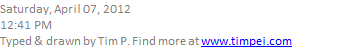Monotonic sequence theorem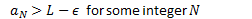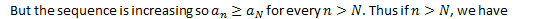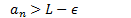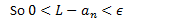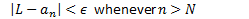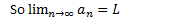Q.E.D.Geometric series

The geometric series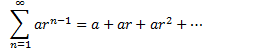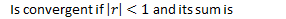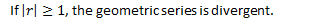Comparison Test

Let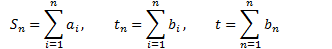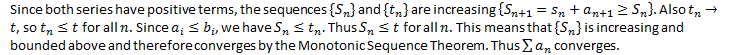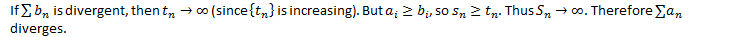Absolute convergence implies convergence

Observe that the inequality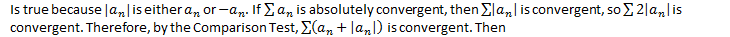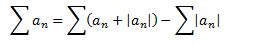Is the difference of two convergent series and is therefore convergent.

Created by Tim Pei with Microsoft OneNote 2010
One place for all your notes and information